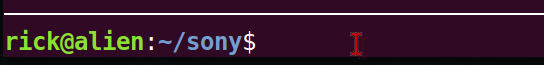{{ message }}

Instantly share code, notes, and snippets.

# rougier/progress_bar.py

Created Jan 25, 2016
A progress bar using unicode character for smoother display
This file contains bidirectional Unicode text that may be interpreted or compiled differently than what appears below. To review, open the file in an editor that reveals hidden Unicode characters. Learn more about bidirectional Unicode characters
 # ----------------------------------------------------------------------------- # Copyright (c) 2016, Nicolas P. Rougier # Distributed under the (new) BSD License. # ----------------------------------------------------------------------------- import sys, math def progress(value, length=40, title = " ", vmin=0.0, vmax=1.0): """ Text progress bar Parameters ---------- value : float Current value to be displayed as progress vmin : float Minimum value vmax : float Maximum value length: int Bar length (in character) title: string Text to be prepend to the bar """ # Block progression is 1/8 blocks = ["", "▏","▎","▍","▌","▋","▊","▉","█"] vmin = vmin or 0.0 vmax = vmax or 1.0 lsep, rsep = "▏", "▕" # Normalize value value = min(max(value, vmin), vmax) value = (value-vmin)/float(vmax-vmin) v = value*length x = math.floor(v) # integer part y = v - x # fractional part base = 0.125 # 0.125 = 1/8 prec = 3 i = int(round(base*math.floor(float(y)/base),prec)/base) bar = "█"*x + blocks[i] n = length-len(bar) bar = lsep + bar + " "*n + rsep sys.stdout.write("\r" + title + bar + " %.1f%%" % (value*100)) sys.stdout.flush() # ----------------------------------------------------------------------------- if __name__ == '__main__': import time for i in range(1000): progress(i, vmin=0, vmax=999) time.sleep(0.0025) sys.stdout.write("\n")

###marc1n commented Sep 29, 2017

 Cool! Instead of: `````` base = 0.125 prec = 3 i = int(round(base*math.floor(float(y)/base),prec)/base) `````` you can write a simpler expression: `i = int(round(y*8))`

###sebassdc commented Nov 27, 2017

 This is awesome! I've made myself a port to Javascript (es6) with a little add (progresive option): ```//@ts-check const progress = ({ value, length=40, title = " ", vmin=0.0, vmax=1.0, progressive = false }) => { // Block progression is 1/8 const blocks = ["", "▏","▎","▍","▌","▋","▊","▉","█"] const lsep = "▏", rsep = "▕" // Normalize value const normalized_value = (Math.min(Math.max(value, vmin), vmax)-vmin)/Number(vmax-vmin) const v = normalized_value * length const x = Math.floor(v) // integer part const y = v - x // fractional part const i = Math.round(y*8) const bar = Array(x).fill("█").join("") + blocks[i] const remaining = Array(length - bar.length).fill(" ").join("") return `\${lsep}\${bar}\${!progressive ? remaining : ""}\${rsep} \${(Math.round(normalized_value * 100 * 100) / 100)}%` } let prevStr = "" for (let i = 0; i < 1000; i++) { prevStr = Array(prevStr.length).fill('\b').join('') + progress({value: i, vmin: 0, vmax: 999}) process.stderr.write(prevStr) } prevStr = "" for (let i = 0; i < 1000; i++) { prevStr = Array(prevStr.length).fill('\b').join('') + progress({value: i, vmin: 0, vmax: 999, progressive: true}) process.stderr.write(prevStr) }```

###WinEunuuchs2Unix commented Dec 31, 2020 • edited Loading

 I wonder if this chokes on Python 2.7.12 or if I copied script wrong? I get this error: ``````Traceback (most recent call last): File "./progress_bar2", line 54, in progress(i, vmin=0, vmax=999) File "./progress_bar2", line 41, in progress bar = "█"*x + blocks[i] TypeError: can't multiply sequence by non-int of type 'float' `````` I did add two lines to the top of the script though: ``````#!/usr/bin/env python # -*- coding: utf-8 -*- `````` Any thoughts?

###rougier commented Dec 31, 2020

 `int(x)` instead of `x` maybe.

###WinEunuuchs2Unix commented Jan 1, 2021 • edited Loading

 @rougler Thanks for the quick reply. I've actually went with a bash solution to the progress bar.The code is here